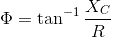# MCQs on Analog Electronics

##### Page 7 of 33. Go to page 1 2 3 4 5 6 7 8 9 10 11 12 13 14 15 16 17 18 19 20 21 22 23 24 25 26 27 28 29 30 31 32 33
01․ When P-type substance is connected with positive and N-type is connected with negative terminal then P-N junction diode is in ....................
Reverse biased
Forward biased
No biased
None of the above

When a diode is connected in a forward biased condition, a negative voltage is applied to the N-type material and a positive voltage is applied to the P-type material. The holes and electrons are repelled by the battery terminal to overcome the junction and current conduction starts.

02․ What is the condition if no external potential energy is applied to the PN junction then diode ?
Zero Bias
Forward biased
Reversed biased
None of the above

When a diode is connected in a zero Bias condition, no external potential energy is applied to the PN junction. It is also called unbiased condition.

03․ If temperature increases then conductivity of a semiconductor is ...................
Decreases
Increases
Constant
None of the above

The electrical conductivity of a semiconductor material increases with increasing temperature. Due to increase in temperature more electron-hole pair are generated and hence conductivity increases.

04․ Which is the factor on which total number of electrons in an atom depends ?
atomic size
atomic weight
atomic number
None of the above

Number of electrons is same as no of protons. The atomic number is the number of protons in the nucleus of an atom. Total number of electrons are determined by atomic number and it is unique for every element.

05․ What is mean by the outermost orbit of an atom ?
Valence orbit
Conduction band
Energy band
None of the above

In any atom, the outermost orbit is called valence orbit or valance band. The number of valence electrons in an atom is the number of electrons present in the outermost orbit or outermost shell of the atom.

06․ What is an energy gap?
Energy band in which electrons can move freely
Energy level at which an electron can exist
Space between two orbital shells
None of the above

The difference of energy between the bottom of the conduction band and the top of the valence band. Energy gap is the space between these two orbital shells. For insulator and semiconductor the energy gap is present but in metal the conduction band and valance band they overlap with each other.

07․ When were the first solid state devices manufactured ?
1960
1950
1970
1940

After a lot of development and success from cats whisker from 1906, silicon solid-state devices were introduced into the electronics industry early in 1960 for commercial purpose.

08․ In a RC phase shift oscillator, the minimum number of R-C networks to be connected in cascade will be
one.
two.
three.
four.

RC phase shift oscillator is used to invert the input for 180° phase difference. The single R-C network gives the 60° phase shift. Hence, we need three set of R-C network which have to be connected in cascade (series). The value of R and C can be chosen by the formula,.

09․ Assume that diode D1, D2 in figure are ideal diodes. The value of current is mcq-diagram
0 mA.
0.5 mA.
1 mA.
2 mA.

Current will pass through the simplest path or low resistance path. D1 becomes forward biased and D2 becomes reverse biased. Therefore current I = 0 mA.

10․ What is the range of energy band gap of semiconductors ?
1-3 ev
0 ev
10-15 ev
14-15 ev

Energy band gap size for semiconductors is in the range 1-3 ev. Example silicon 1.1 ev.

<<<56789>>>